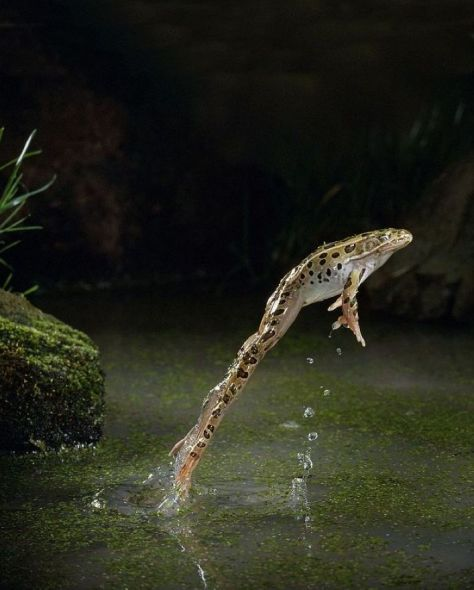人类为什么不能水上漂？ — 解析2013年搞笑Nobel物理奖精选

2013年搞笑Nobel物理学奖授予了Alberto  E. Minetti等人，因为他们去年在Plos One上发表的论文：Humans Running in Place on Water at Simulated Reduced Gravity， Alberto  E. Minetti et al,  PLoS ONE, 7, 37300(2013). http://www.plosone.org/article/info:doi/10.1371/journal.pone.0037300

1. 背景介绍2. 重力和人是否可以浮水面的关系

3. 哪些因素决定了是否可以水上漂?

• 质量

• 踩踏速度和频率 (和体力有关)

• 脚和水的接触面积 (这是和尚为什么要在脚下放大木板的原因)

• 重力加速度$g$ (这应该是他们获得搞笑Nobel奖的原因, 正常的人不会这样想问题)

4. 基本原理 (Glsheen and McMahon, Nature, 1996)

$F = ma$

5. 什么时候才能水上漂?  （我自己的总结)

• 一个人的体重为$M$, 那么重力为$Mg$.所以在单位时间内$\delta T$， 重力的冲量为$P = Mg\delta T$.

•  每次人脚踩水面的时候，水给人向上的力为$F_0$, 时间为$\delta T_0$。

• 每次只有一只脚在水中， 同时在空中的时间可以忽略不计，那么在$\delta T$时间内，人踩踏水的频率为$f$

$F_0 \propto Mg f$。

6. 水填充空气腔的时间和重力的关系

$t = 2.285 (r/g)^{1/2}$

$f = 1/2t = 0.219 (g/r)^{-1/2}$

$F_0 \propto M g^{1/2}$,

7. 总结

https://blog.sciencenet.cn/blog-709494-730206.html